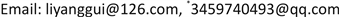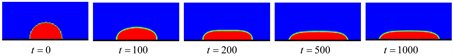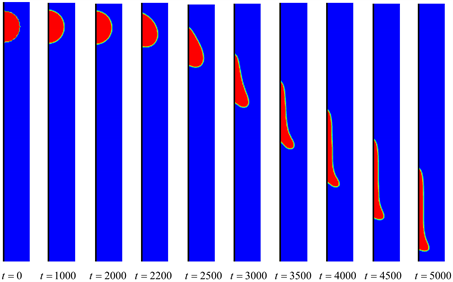﻿ 固壁上液滴动力学的LBM建模与计算 LBM Modeling and Calculation for Droplet Dynamics on Solid Wall

Applied Physics
Vol. 09  No. 05 ( 2019 ), Article ID: 30481 , 5 pages
10.12677/APP.2019.95032

LBM Modeling and Calculation for Droplet Dynamics on Solid Wall

Yanggui Li1,2, Dacheng Liang3*

1School of Mathematics and Statistics, Lingnan Normal University, Zhanjiang Guangdong

2Institute of Synthetic Biology, Shenzhen Institute of Advanced Technology, Chinese Academy of Sciences, Shenzhen Guangdong

3Department of Mathematics, Guangdong Preschool Normal College in Maoming, Maoming GuangdongReceived: May 8th, 2019; accepted: May 21st, 2019; published: May 28th, 2019ABSTRACT

In this paper, the dynamic model for the droplet on the solid wall is studied with Shan-Chen multiphase lattice Boltzmann method. Based on the model, the spreading behavior of droplet on the horizontal solid wall and the slip behavior on the vertical solid wall are simulated.

Keywords:Droplet Dynamics, Lattice Boltzmann Method, Solid-Liquid Interaction, Multiphase Flow

1岭南师范学院，数学与统计学院，广东 湛江

2中国科学院深圳先进技术研究院，合成生物学研究所，广东 深圳

3广东茂名幼儿师范专科学校，数学系，广东 茂名1. 引言

2. 理论与模型

2.1. 格子Boltzmann方法

Boltzmann方程描述了由于其分子的流动和碰撞而导致的流体状态的演化。LBM是通过对Boltzmann方程的相空间进行离散化得到的，其中大量的分子速度被一组有限的速度矢量(晶格)代替  。LBM模型包含三要素：格子结构、流体粒子的离散速度集合、演化方程，其描述了流体粒子分布函数在固定格子上的演化过程

${f}_{i}\left(x+{e}_{i}{\delta }_{i},t+{\delta }_{i}\right)-{f}_{i}\left(x,t\right)={\Omega }_{i}\left(x,t\right)$ (1)

$\rho =m{\sum }_{i}{f}_{i}$ , $\rho u=m{\sum }_{i}{e}_{i}{f}_{i}$ (2)

m为流体粒子质量。

2.2. 多相或多组分格子Boltzmann模型

${f}_{i}^{k}\left(x+{e}_{i}{\delta }_{i},t+{\delta }_{i}\right)-{f}_{i}^{k}\left(x,t\right)=-\frac{{f}_{i}^{k}\left(x,t\right)-{f}_{i}^{k\left(eq\right)}\left(x,t\right)}{{\tau }_{k}}$ (3)

${f}_{0}^{k\left(eq\right)}={\alpha }_{k}{n}_{k}-\frac{2}{3}{n}_{k}{u}_{k}^{eq}\cdot {u}_{k}^{eq}$ (4)

${f}_{i}^{k\left(eq\right)}=\frac{\left(1-{\alpha }_{k}\right){n}_{k}}{5}+\frac{1}{3}{n}_{k}\left({e}_{i}\cdot {u}_{k}^{eq}\right)+\frac{1}{2}{n}_{k}{\left({e}_{i}\cdot {u}_{k}^{eq}\right)}^{2}-\frac{1}{6}{n}_{k}{u}_{k}^{eq}{u}_{k}^{eq}\text{\hspace{0.17em}}\text{\hspace{0.17em}}\text{\hspace{0.17em}}\text{\hspace{0.17em}}\text{\hspace{0.17em}}\text{\hspace{0.17em}}i=1,2,3,4$ (5)

${f}_{i}^{k\left(eq\right)}=\frac{\left(1-{\alpha }_{k}\right){n}_{k}}{20}+\frac{1}{12}{n}_{k}\left({e}_{i}\cdot {u}_{k}^{eq}\right)+\frac{1}{8}{n}_{k}{\left({e}_{i}\cdot {u}_{k}^{eq}\right)}^{2}-\frac{1}{24}{n}_{k}{u}_{k}^{eq}{u}_{k}^{eq}\text{\hspace{0.17em}}\text{\hspace{0.17em}}\text{\hspace{0.17em}}\text{\hspace{0.17em}}i=5,6,7,8$ (6)

${e}_{i}=\left\{\begin{array}{l}0\text{\hspace{0.17em}}\text{\hspace{0.17em}}\text{\hspace{0.17em}}\text{\hspace{0.17em}}\text{\hspace{0.17em}}\text{\hspace{0.17em}}\text{\hspace{0.17em}}\text{\hspace{0.17em}}i=0\\ \left(\mathrm{cos}\frac{\left(i-1\right)\text{π}}{2},\mathrm{sin}\frac{\left(i-1\right)\text{π}}{2}\right)\text{\hspace{0.17em}}\text{\hspace{0.17em}}\text{\hspace{0.17em}}\text{\hspace{0.17em}}\text{\hspace{0.17em}}\text{\hspace{0.17em}}i=1,2,3,4\\ \sqrt{\text{2}}\left(\text{cos}\left[\frac{\left(i-5\right)\text{π}}{2}+\frac{\text{π}}{4}\right],\mathrm{sin}\left[\frac{\left(i-5\right)\text{π}}{2}+\frac{\text{π}}{4}\right]\right)\text{\hspace{0.17em}}\text{\hspace{0.17em}}\text{\hspace{0.17em}}\text{\hspace{0.17em}}\text{\hspace{0.17em}}i=5,6,7,8\end{array}$ (7)

${\alpha }_{k}$ 是一个自由参数，它与纯组分k区域的声速有关，其关系式为 ${\left({c}_{s}^{k}\right)}^{2}=\frac{3}{5}\left(1-{\alpha }_{k}\right)$${n}_{k}={\sum }_{i}{f}_{i}^{k}$ 是k组分的数密度。k组分的质量密度 ${\rho }_{k}$ 定义为 ${\rho }_{k}={m}_{k}{n}_{k}={m}_{k}{\sum }_{i}{f}_{i}^{k}$ ，组分k的流体速度 ${u}_{k}$ 定义为 ${\rho }_{k}{u}_{k}={m}_{k}{\sum }_{i}{e}_{i}{f}_{i}^{k}$ ，其中 ${m}_{k}$ 是k组分的分子质量。参数 ${u}_{k}^{eq}$ 由如下关系式确定：

${\rho }_{k}{u}_{k}^{eq}={\rho }_{k}{u}^{\prime }+{\tau }_{k}{F}_{k}$ (8)

${u}^{\prime }=\left(\underset{k=1}{\overset{s}{\sum }}\frac{{\rho }_{k}{u}_{k}}{{\tau }_{k}}\right)/\left(\underset{k=1}{\overset{s}{\sum }}\frac{{\rho }_{k}}{{\tau }_{k}}\right)$ (9)

${F}_{1k}\left(x\right)=-{\Psi }_{k}\left(x\right)\underset{{x}^{\prime }}{\sum }\underset{k=1}{\overset{s}{\sum }}{G}_{k\stackrel{¯}{k}}\left(x,{x}^{\prime }\right){\Psi }_{\stackrel{¯}{k}}\left({x}^{\prime }\right)\left(x-{x}^{\prime }\right)$ (10)

${G}_{k\stackrel{¯}{k}}\left(x,{x}^{\prime }\right)=\left\{\begin{array}{l}{g}_{k\stackrel{¯}{k}},\text{\hspace{0.17em}}\text{\hspace{0.17em}}\text{\hspace{0.17em}}\text{\hspace{0.17em}}\text{\hspace{0.17em}}\text{\hspace{0.17em}}\text{\hspace{0.17em}}|x-{x}^{\prime }|=1\\ {g}_{k\stackrel{¯}{k}}/4,\text{\hspace{0.17em}}\text{\hspace{0.17em}}\text{\hspace{0.17em}}|x-{x}^{\prime }|=\sqrt{2}\\ 0,\text{\hspace{0.17em}}\text{\hspace{0.17em}}\text{\hspace{0.17em}}\text{\hspace{0.17em}}\text{\hspace{0.17em}}\text{\hspace{0.17em}}\text{\hspace{0.17em}}\text{\hspace{0.17em}}\text{\hspace{0.17em}}\text{\hspace{0.17em}}\text{\hspace{0.17em}}\text{\hspace{0.17em}}其它\end{array}$ (11)

${F}_{2k}={\rho }_{k}g={m}_{k}{n}_{k}g$ (12)

2.3. 流体与固壁之间的相互作用

${F}_{3k}\left(x\right)=-{n}_{k}\left(x\right)\underset{{x}^{\prime }}{\sum }{g}_{kw}{n}_{w}\left({x}^{\prime }\right)\left({x}^{\prime }-x\right)$ (13)

$\frac{\partial \rho }{\partial t}+\nabla \cdot \left(\rho u\right)=0$ (14)

$\rho \left[\frac{\partial u}{\partial t}+\left(u\cdot \nabla \right)u\right]=-\nabla p+\nabla \cdot \left[\rho \nu \left(\nabla u+u\nabla \right)\right]+\rho g$ (15)

3. 数值模拟Figure 1. Evolution of droplet spreading on solid wallFigure 2. Evolution of droplet slipping on solid wall

4. 总结

LBM Modeling and Calculation for Droplet Dynamics on Solid Wall[J]. 应用物理, 2019, 09(05): 269-273. https://doi.org/10.12677/APP.2019.95032

1. 1. Shan, X.W. and Chen, H.D. (1993) Lattice Boltzmann Model for Simulation Flows with Multiple Phases and Compo-nents. Physical Review E, 47, 1815-1993. https://doi.org/10.1103/PhysRevE.47.1815

2. 2. Kang, Q.J., Zhang, D. and Chen, S. (2002) Displacement of a Two-Dimensional Immiscible Droplet in a Channel. Physics of Fluids, 14, 3203-3214. https://doi.org/10.1063/1.1499125

3. 3. Xing, X.Q., Butler, D.L. and Yang, C. (2007) Lattice Boltz-mann-Based Single-Phase Method for Free Surface Tracking of Droplet Motions. International Journal for Numerical Methods in Fluidss, 53, 333-351. https://doi.org/10.1002/fld.1282

4. 4. Tanaka, Y., Washio, Y., Yoshino, M. and Hirata, T. (2011) Numerical Sim-ulation of Dynamic Behavior of Droplet on Solid Surface by the Two-Phase Lattice Boltzmann Method. Computers & Fluids, 40, 68-78. https://doi.org/10.1016/j.compfluid.2010.08.007

5. 5. Wu, J., Huang, J.J. and Yan, W.W. (2015) Lattice Boltzmann Investigation of Droplets Impact Behaviors onto a Solid Substrate. Colloids and Surfaces A: Physicochemical and Engineering Aspects, 484, 318-328. https://doi.org/10.1016/j.colsurfa.2015.07.043

6. 6. Mousavi Tilehboni, S.E., Fattahi, E., Afrouzi, H.H. and Farhadi, M. (2015) Numerical Simulation of Droplet Detachment from Solid Walls under Gravity Force Using Lattice Boltzmann Method. Journal of Molecular Liquids, 212, 544-556. https://doi.org/10.1016/j.molliq.2015.10.007

7. 7. Raman, K.A., Jaiman, R.K., Lee, T.S. and Low, H.T. (2016) Lattice Boltzmann Study on the Dynamics of Successive Droplets Impact on a Solid Surface. Chemical Engineering Science, 145, 181-195. https://doi.org/10.1016/j.ces.2016.02.017

8. NOTES

*通讯作者。